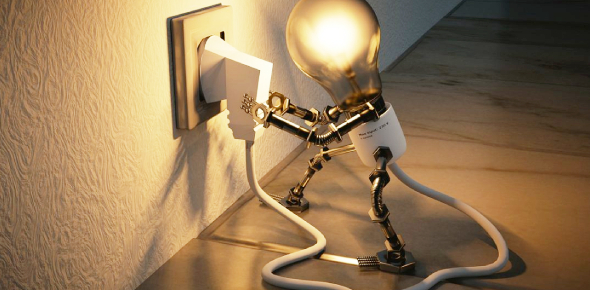# Toughest Test On Electricity: Trivia Quiz

50 Questions | Total Attempts: 46Settings.

Related Topics
• 1.
In the mid-1800's a British scientist named ___________________________ discovered that he could use magnets to cause electric current to flow through a conductor.
• A.

Alessandro Volta

• B.

Eli Whitney

• C.

• D.

Andre Ampere

• 2.
Friction between two objects which causes an electrical charge is known as _______________ .
• A.

Photoelectricity

• B.

Static electricity

• C.

Magnet induction

• D.

Piezoelectricity

• E.

Thermoelectricity

• 3.
What are the two types of electrical charges?
• A.

Short and long

• B.

Positive and negative

• C.

High and low

• D.

Slow and fast

• 4.
What sort of charge does an electron have?
• A.

Positive

• B.

Negative

• C.

Neutral

• D.

• 5.
You have a balloon and some hair, how do you create static electricity?
• A.

Blow up the balloon and comb your hair

• B.

Tie the balloon to the hair

• C.

Rub the balloon against your hair

• D.

Wear the balloon as a hair tie

• 6.
AC is one form of electrical current, what is the other common form?
• A.

BC

• B.

CC

• C.

DC

• D.

EC

• 7.
Why should you never directly connect the negative and positive terminals of a battery?
• A.

It will short circuit

• B.

You will get a shock

• C.

The battery will turn blue

• D.

The battery will disappear

• 8.
What happens when a switch is turned off?
• A.

Electricity stops flowing from the switch and therefore the circuit is broken, providing no electricity to the object being powered

• B.

Electricity is able to flow through the circuit powering an electrical device

• C.

Electricity is not able to flow through the circuit but it can still power an electrical device

• D.

Electricity is able to flow through the circuit but it cannot power an electrical device

• 9.
Watt-hours is a measurement of:
• A.

Power

• B.

Energy potential

• C.

Current

• D.

Resistance

• 10.
Voltage is the measurement of:
• A.

Current

• B.

Power

• C.

Energy potential

• D.

Resistance

• 11.
The type of current found in our homes is
• A.

Alternating current

• B.

Director current

• 12.
Is this a series or parallel circuit?
• A.

Parallel

• B.

Series

• 13.
Will this light bulb work under this wiring arrangement?
• A.

Yes

• B.

No

• 14.
Current coming from a solar panel is:
• A.

Alternating current

• B.

Direct current

• 15.
What happens when two similar charged object are brought close to  each other?
• A.

They will attract.

• B.

They will repel.

• C.

They will attack.

• D.

They will do nothing.

• 16.
__________________ electricity results when electrons are able to move along a conductor.
• A.

Wire

• B.

Doorknob

• C.

Static

• D.

Current

• 17.
What kind of a charge do you have when the number of negative charges equals the number of positive?
• A.

No Charge

• B.

Positive charge

• C.

Negative Charge

• D.

Imbalance

• 18.
What is a conductor of electricity?
• A.

Something that electricity can't move through

• B.

Something that makes electricity stop

• C.

Something that electricity can move through

• D.

Something that makes electricity repel magnets

• 19.
What do most power plants use to make electricity?
• A.

Heat.

• B.

Light.

• C.

Air.

• D.

Water.

• 20.
Why are electrical wires made of metal?
• A.

Because metal is cheap

• B.

Because metal is a good conductor

• C.

So they can bend easily

• D.

To insulate

• 21.
Why is there usually a plastic coating around a metal wire?
• A.

To keep the wire warm

• B.

So it looks nice

• C.

To conduct electricity

• D.

To insulate the wire from other things

• 22.
If you add an extra battery to a circuit containing a bulb, how will this affect the bulb?
• A.

The bulb will turn off

• B.

There will be no change to the bulb

• C.

The bulb will get brighter

• D.

The bulb will get dimmer

• 23.
This is a Parallel Circuit?
• A.

True

• B.

False

• 24.
In this circuit the bulbs would be on?
• A.

True

• B.

False

• 25.
Materials that do not allow electricity to flow are called ?
• A.

Circuit

• B.

Insulator

• C.

Conductor